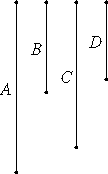# Proposition 13

If four numbers are proportional, then they are also proportional alternately.

Let the four numbers A, B, C, and D be proportional, so that A is to B as C is to D.I say that they are also proportional alternately, so that A is to C as B is to D.

VII.Def.20

Since A is to B as C is to D, therefore, A is the same part or parts of B as C is of D.

VII.10

Therefore, alternately, A is the same part or parts of C as B is of D.

VII.Def.20

Therefore A is to C as B is to D.

Therefore, if four numbers are proportional, then they are also proportional alternately.

Q.E.D.

## Guide

This is the numerical analogue of proposition V.16 for magnitudes. It says that

if a : b = c : d, then a : c = b : d.

This proposition is used frequently in Books VII through IX starting with the next proposition.